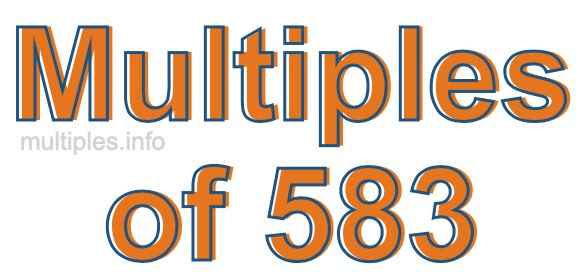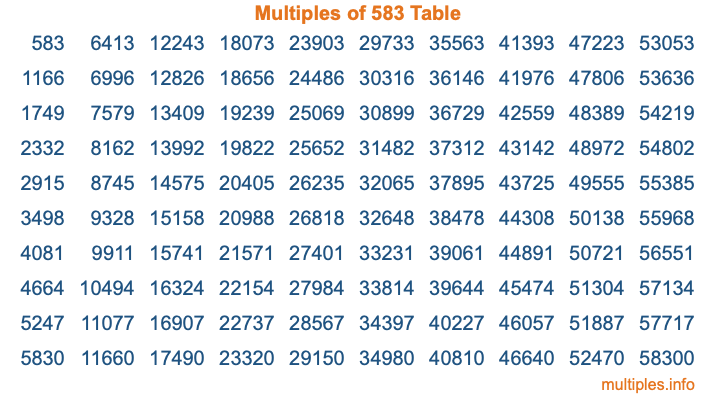Multiples of 583Welcome to the Multiples of 583 page. Here we will first teach you everything you will ever need to know about the multiples of 583, and then give you a study guide summary of everything we taught you to make sure you remember it all. Use this page to look up facts and learn information about the multiples of 583. This page will make you a multiples of five hundred eighty-three expert!

Definition of Multiples of 583
Multiples of 583 are all the numbers that when divided by 583 equal an integer. Each of the multiples of 583 are called a multiple. A multiple of 583 is created by multiplying 583 by an integer.

Therefore, to create a list of multiples of 583, you start with 1 multiplied by 583, then 2 multiplied by 583, then 3 multiplied by 583, and so on for as long as you want. Thus, the list of the first five multiples of 583 is 583, 1166, 1749, 2332, and 2915. To see a larger list of multiples of 583, see the printable image of Multiples of 583 further down on this page. We also have a category where you can choose any nth multiple of 583.

Multiples of 583 Checker
The Multiples of 583 Checker below checks to see if any number of your choice is a multiple of 583. In other words, it checks to see if there is any number (integer) that when multiplied by 583 will equal your number. To do that, we divide your number by 583. If the the quotient is an integer, then your number is a multiple of 583.

Is  a multiple of 583?

Least Common Multiple of 583 and ...
A Least Common Multiple (LCM) is the lowest multiple that two or more numbers have in common. This is also called the smallest common multiple or lowest common multiple and is useful to know when you are adding our subtracting fractions. Enter one or more numbers below (583 is already entered) to find the LCM.

Check out our LCM Calculator if you need more details about the Least Common Multiple or if you need the LCM for different numbers for adding and subtraction fractions.

nth Multiple of 583
As we stated above, 583 is the first multiple of 583, 1166 is the second multiple of 583, 1749 is the third multiple of 583, and so on. Enter a number below to find the nth multiple of 583.

th multiple of 583

Multiples of 583 vs Factors of 583
583 is a multiple of 583 and a factor of 583, but that is where the similarities end. All postive multiples of 583 are 583 or greater than 583. All positive factors of 583 are 583 or less than 583.

Below is the beginning list of multiples of 583 and the factors of 583 so you can compare:

Multiples of 583: 583, 1166, 1749, 2332, 2915, etc.

Factors of 583: 1, 11, 53, 583

As you can see, the multiples of 583 are all the numbers that you can divide by 583 to get a whole number. The factors of 583, on the other hand, are all the whole numbers that you can multiply by another whole number to get 583.

It's also interesting to note that if a number (x) is a factor of 583, then 583 will also be a multiple of that number (x).

Multiples of 583 vs Divisors of 583
The divisors of 583 are all the integers that 583 can be divided by evenly. Below is a list of the divisors of 583.

Divisors of 583: 1, 11, 53, 583

The interesting thing to note here is that if you take any multiple of 583 and divide it by a divisor of 583, you will see that the quotient is an integer.

Multiples of 583 Table
Below is an image of the first 100 multiples of 583 in a table. The table is in chronological order, column by column. The first column has the first ten multiples of 583, the second column has the next ten multiples of 583, and so on.The Multiples of 583 Table is also referred to as the 583 Times Table or Times Table of 583. You are welcome to print out our table for your studies.

Negative Multiples of 583
Although not often discussed or needed in math, it is worth mentioning that you can make a list of negative multiples of 583 by multiplying 583 by -1, then by -2, then by -3, and so on, to get the following list of negative multiples of 583:

-583, -1166, -1749, -2332, -2915, etc.

Multiples of 583 Summary
Below is a summary of important Multiples of 583 facts that we have discussed on this page. To retain the knowledge on this page, we recommend that you read through the summary and explain to yourself or a study partner why they hold true.

There are an infinite number of multiples of 583.

A multiple of 583 divided by 583 will equal a whole number.

583 divided by a factor of 583 equals a divisor of 583.

The nth multiple of 583 is n times 583.

The largest factor of 583 is equal to the first positive multiple of 583.

583 is a multiple of every factor of 583.

583 is a multiple of 583.

A multiple of 583 divided by a divisor of 583 equals an integer.

583 divided by a divisor of 583 equals a factor of 583.

Any integer times 583 will equal a multiple of 583.

Multiples of a Number
Here you can get the multiples of another number, all with the same attention to detail as we did for multiples of 583 on this page.

Multiples of
Multiples of 584
Did you find our page about multiples of five hundred eighty-three educational? Do you want more knowledge? Check out the multiples of the next number on our list!

Copyright  |   Privacy Policy  |   Disclaimer  |   Contact